International
Tables for
Crystallography
Volume B
Reciprocal space
Edited by U. Shmueli

International Tables for Crystallography (2006). Vol. B. ch. 2.1, pp. 197-198   | 1 | 2 |

## Section 2.1.6.2. Distribution of ratios

U. Shmuelia* and A. J. C. Wilsonb

aSchool of Chemistry, Tel Aviv University, Tel Aviv 69 978, Israel, and bSt John's College, Cambridge, England
Correspondence e-mail:  ushmueli@post.tau.ac.il

#### 2.1.6.2. Distribution of ratios

| top | pdf |

Ratios like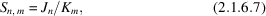where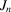is given by equation (2.1.6.1),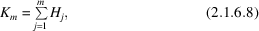and the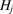's are the intensities of a set of reflections (which may or may not overlap with those included in), are used in correlating intensities measured under different conditions. They arise in correlating reflections on different layer lines from the same or different specimens, in correlating the same reflections from different crystals, in normalizing intensities to the local average or to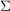, and in certain systematic trial-and-error methods of structure determination (see Rabinovich & Shakked, 1984, and references therein). There are three main cases:

 (i)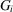and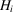refer to the same reflection; for example, they might be the observed and calculated quantities for thereflection measured under different conditions or for different crystals of the same substance; or (ii)andare unrelated; for example, the observed and calculated values for thereflection for a completely wrong trial structure, of values for entirely different reflections, as in reducing photographic measurements on different layer lines to the same scale; or (iii) the's are a subset of the's, so that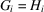for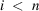and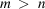.

Aside from the scale factor, in case (i)andwill differ chiefly through relatively small statistical fluctuations and uncorrected systematic errors, whereas in case (ii)the differences will be relatively large because of the inherent differences in the intensities. Here we are concerned only with cases (ii)and (iii); the practical problems of case (i)are postponed to IT C (2004).

There is little in the crystallographic literature concerning the probability distribution of sums like (2.1.6.1)or ratios like (2.1.6.7); certain results are reviewed by Srinivasan & Parthasarathy (1976, ch. 5), but with a bias toward partially related structures that makes it difficult to apply them to the immediate problem.

In case (ii)(andindependent), acentric distribution, Table 2.1.5.1gives the distribution of the ratio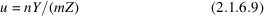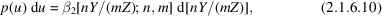where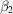is a beta distribution of the second kind, Y is given by equation (2.1.6.2)and Z by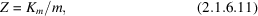where n is the number of intensities included in the numerator and m is the number in the denominator. The expected value of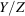is then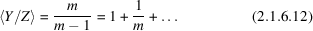with varianceOne sees thatis a biased estimate of the scaling factor between two sets of intensities and the bias, of the order of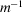, depends only on the number of intensities averaged in the denominator. This may seem odd at first sight, but it becomes plausible when one remembers that the mean of a quantity is an unbiased estimator of itself, but the reciprocal of a mean is not an unbiased estimator of the mean of a reciprocal. The mean exists only if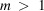and the variance only for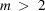.

In the centric case, the expression for the distribution of the ratio of the two means Y and Z becomes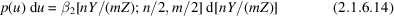with the expected value ofequal to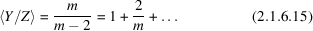and with its variance equal to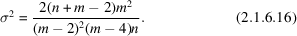For the same number of reflections, the bias in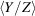and the variance for the centric distribution are considerably larger than for the acentric. For both distributions the variance of the scaling factor approaches zero when n and m become large. The variances are large for m small, in fact `infinite' if the number of terms averaged in the denominator is sufficiently small. These biases are readily removed by multiplyingby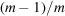or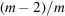. Many methods of estimating scaling factors – perhaps most – also introduce bias (Wilson, 1975; Lomer & Wilson, 1975; Wilson, 1976, 1978c) that is not so easily removed. Wilson (1986a) has given reasons for supposing that the bias of the ratio (2.1.6.7)approximates to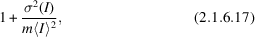whatever the intensity distribution. Equations (2.1.6.12)and (2.1.6.15)are consistent with this.

### ReferencesInternational Tables for Crystallography (2004). Vol. C. Mathematical, physical and chemical tables, edited by E. Prince. Dordrecht: Kluwer Academic Publishers.Google ScholarLomer, T. R. & Wilson, A. J. C. (1975). Scaling of intensities. Acta Cryst. B31, 646–647.Google ScholarRabinovich, D. & Shakked, Z. (1984). A new approach to structure determination of large molecules by multi-dimensional search methods. Acta Cryst. A40, 195–200.Google ScholarSrinivasan, R. & Parthasarathy, S. (1976). Some statistical applications in X-ray crystallography. Oxford: Pergamon Press. Google ScholarWilson, A. J. C. (1975). Effect of neglect of dispersion on apparent scale and temperature factors. In Anomalous scattering, edited by S. Ramaseshan & S. C. Abrahams, pp. 325–332. Copenhagen: Munksgaard.Google ScholarWilson, A. J. C. (1976). Statistical bias in least-squares refinement. Acta Cryst. A32, 994–996.Google ScholarWilson, A. J. C. (1978c). Statistical bias in scaling factors: Erratum. Acta Cryst. B34, 1749.Google ScholarWilson, A. J. C. (1986a). Distributions of sums and ratios of sums of intensities. Acta Cryst. A42, 334–339.Google Scholar